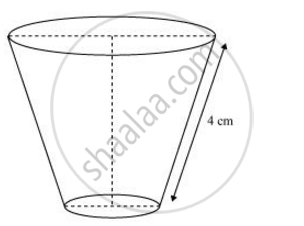Share

# The slant height of a frustum of a cone is 4 cm and the perimeters (circumference) of its circular ends are 18 cm and 6 cm. find the curved surface area of the frustum. - Mathematics

Course

#### Question

The slant height of a frustum of a cone is 4 cm and the perimeters (circumference) of its circular ends are 18 cm and 6 cm. find the curved surface area of the frustum.

#### Solution 1Perimeter of upper circular end of frustum = 18 cm

r1 =18

r1 = 9/π

Perimeter of lower end of frustum = 6 cm

r2 = 6

r2 = 3/π

Slant height (l) of frustum = 4 cm

CSA of frustum = π (r1 + r2l

=pi(9/pi+3/pi)4

= 12x4

= 48 cm2

Therefore, the curved surface area of the frustum is 48 cm2.

#### Solution 2

We have,

Perimeter of upper end, C=18cm,

Perimeter of lower end, c=6 cm and

Slant Height, l=4 cm

Let the radius of upper end be R and the radius of lower end be r.

As, C = 18 cm

⇒2πR = 18

=> R = 18/(2pi)

=> R = 9/picm

Similary c = 6 cm

=> r = 6/(2pi)

=> r = 3/pi cm

Curved surface area of the frustum=π(R + r)l

=pi(9/pi + 3/pi) xx 4

= pi xx 12/pi xx 4

= 48 cm^2

Hence, the curved surface area of the frustum is 48 cm2

Is there an error in this question or solution?

#### APPEARS IN

NCERT Solution for Mathematics Textbook for Class 10 (2019 (Latest))
Chapter 13: Surface Areas and Volumes
Ex. 13.4 | Q: 2 | Page no. 257
RD Sharma Solution for Class 10 Maths (2018 (Latest))
Chapter 14: Surface Areas and Volumes
Ex. 14.3 | Q: 3 | Page no. 78

#### Video TutorialsVIEW ALL 

Solution The slant height of a frustum of a cone is 4 cm and the perimeters (circumference) of its circular ends are 18 cm and 6 cm. find the curved surface area of the frustum. Concept: Frustum of a Cone.
S